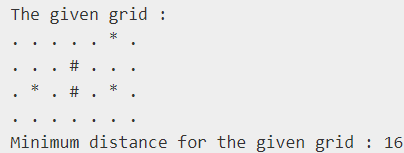## Introduction

The first thing we need to make sure of before solving any DP with Bitmasking problem is that the constraints must be small because this approach takes exponential time and space.

Let us now discuss what is a Bitmask.
Bitmask is a Binary Number that stores some value inside it. Generally, we use Bitmask to memoize a recursive solution that represents the things we have already considered or visited.

Let us understand this with an example of the problem we will discuss further.

There is a salesman who wants to visit all the N cities once. Here the bitmask will be a Binary number and it will represent how many cities we have already visited when we are at a particular recursive call. The ith bit number which is set to 1 will represent that we have visited the ith city.
We maintain this Bitmask value and using this Bitmask value we try to memoize our recursive code.

Now, let us discuss the TSP problem.

We are given a 2-D array of characters, including ‘*’, ‘.’, and ‘#’.
*’ represents the Houses.

We are currently at (0, 0) index and we need to visit all the houses only once and return back to our initial position (0, 0) at the end of the journey. We need to find the minimum distance to visit all the houses.

## Approach

Our first step will be to calculate the shortest distance between the two houses. For this, we will run BFS from each house and find its minimum distance from each cell considering it as the source cell.

We will store the above result in a table of size O(Grid_size * N) (where ‘N’ is the number of houses) so that we don’t need to calculate it each time.

Now, we will try out all the combinations of traveling the houses and determine the minimum distance between them.
We will be using DP with Bitmasking to store the result for different states to reuse them.

Let us define the DP with Bitmasking state for the same.
DP[index][mask]: It will represent the minimum distance covered to reach the (index)th house after traveling all the houses stored in the mask. All the bits which are set to 1 in the mask are visited by us in the current state.

As soon as the mask has all the bits set to 1((1 << N) - 1), that means we have visited all the cities and it is our answer.

At any state (index, mask), if all the bits are not set in the mask, we will visit the next house which is not already visited.

Hence the transition of DP with Bitmasking would look like as follows:

Refer to the below implementation of the above approach.

OutputTime Complexity: There will be a maximum of N * (2 ^ N) states and for each state, we will be traversing all the N houses. Hence, the overall time complexity will be O(N ^ 2 * 2 ^ N).

Space Complexity: We are maintaining a DP table of size (N * 2 ^ N) which is a dominating term. Hence, the Space Complexity is O(N * 2^N).

## FAQs

1. What is the Time and Space complexity of the approach used?
Time Complexity: There will be a maximum of N * (2 ^ N) states and for each state, we will be traversing all the N houses. Hence, the overall time complexity will be O((N ^ 2) * 2 ^ N).
Space Complexity: We are maintaining a DP table of size (N * (2 ^ N)) which is a dominating term. Hence, the Space Complexity is O(N * (2 ^ N)).

It will represent the minimum distance covered to reach the (index)th house after traveling all the houses stored in the mask. All the bits which are set to 1 in the mask are visited by us in the current state.

## Key Takeaways

In this blog, we have covered the following things:

1. We first discussed the DP with Bitmasking approach to solve this problem.
2. Then we discussed the code, time, and space complexity of the approach used.

If you want to learn more about DP with Bitmasking and want to practice some questions which require you to take your basic knowledge on Dynamic Programming a notch higher, then you can visit our Guided Path for Dynamic Programming

Until then, All the best for your future endeavors, and Keep Coding.